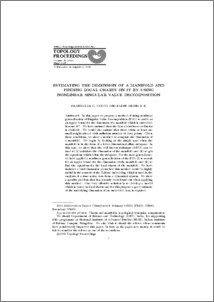# Estimating the dimension of a manifold and finding local charts on it by using nonlinear single value decomposition

Vaidya, Prabhakar G and Anand PS, Sajini (2014) Estimating the dimension of a manifold and finding local charts on it by using nonlinear single value decomposition. Topology Proceedings, 43. pp. 277-291. ISSN 0146-4124 (Print), 2331-1290 (Online)Preview
PDF
VaidyaSajini.pdf - Published Version

Download (174kB)
ContributionNameEmail
Abstract: In this paper we propose a method of using nonlinear generalization of Singular Value Decomposition (SVD) to arrive at an upper bound for the dimension of a manifold which is embedded in some RN. We have assumed that the data about its co-ordinates is available. We would also assume that there exists at least one small neighborhood with sufficient number of data points. Given these conditions, we show a method to compute the dimension of a manifold. We begin by looking at the simple case when the manifold is in the form of a lower dimensional affine subspace. In this case, we show that the well known technique of SVD can be used to (i) calculate the dimension of the manifold and (ii) to get the equations which define the subspace. For the more general case, we have applied a nonlinear generalization of the SVD (i) to search for an upper bound for the dimension of the manifold and (ii) to find the equations for the local charts of the manifold. We have included a brief discussion about how this method would be highly useful in the context of the Takens’ embedding which is used in the analysis of a time series data from a dynamical system. We show a specific problem that has recently been found out when applying this method. One very effective solution is to develop a model which is based on local charts and for this purpose a good estimate of the underlying dimension of an embedded data is required. Article Copyright belongs to the Publisher Charts and manifolds; topological dynamics; computation School of Natural and Engineering Sciences > Mathematical Modeling Schools > Natural Sciences and Engineering 20 Mar 2014 05:06 08 May 2015 10:21 http://topology.auburn.edu/tp/ UNSPECIFIED UNSPECIFIED http://eprints.nias.res.in/id/eprint/599

### Actions (login required)View Item